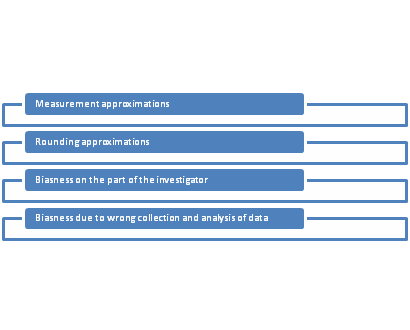# Error in Statistics and its reasons

Definition Error in Statistics:

Error in statistics means the difference between true value and the estimated value. It is different from the mistakes which may be done while making calculations or observations.

Reasons of Error in Statistics:

The errors in statistics may arise due to following reasons:

1. Measurement approximations: For example-height or weight is measured in round figure so there will be difference between observed value and true value.
2. Rounding approximations: For example-values are taken to the nearest hundreds or thousands etc.
3. Biasness on the part of the investigator
4. Biasness due to wrong collection and analysis of data

The reasons of errors are highlighted in form of figure below:Also Read: Types of errors in statistics